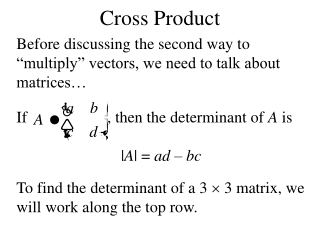DownloadDownload PresentationCross Product

# Cross Product

Download Presentation## Cross Product

- - - - - - - - - - - - - - - - - - - - - - - - - - - E N D - - - - - - - - - - - - - - - - - - - - - - - - - - -
##### Presentation Transcript

1. Cross Product Before discussing the second way to “multiply” vectors, we need to talk about matrices… If , then the determinant of A is |A| = ad – bc To find the determinant of a 3  3 matrix, we will work along the top row.

2. Ex.

3. Def. Let and , then the cross product is This is also called the vector product, since the result is a vector This only makes sense in V3

4. Ex.

5. Thm. The vector a b is orthogonal to a and b. Thm.a b = –(b a) To find the orientation of a b, use your right hand: a = index finger b = three fingers a b = thumb Switching the order of the cross product reverses the orientation

6. Ex. Find a vector perpendicular to the plane that contains the points P(1,4,6), Q(-2,5,-1), and R(1,-1,1).

7. Thm. |a b| = |a| |b| sin θ, where θ is the angle between a and b. Cor. If a b = 0, then a and b are parallel. Thm. The magnitude of a b is the area of the parallelogram formed by a and b.

8. Ex. Find the area of the triangle with vertices P(1,4,6), Q(-2,5,-1), and R(1,-1,1).

9. Properties of the Cross Product • (ca)  b = c(a  b) = a  (cb) • a  (b + c) = a  b + a  c • (a + b)  c = a  c + b  c • a∙ (b c) = (a  b) ∙ c • a (b  c) = (a ∙ c)b – (a ∙ b)c

10. The quantity a ∙ (b  c) is called the scalar triple product. Thm. The volume of the parallelepiped determined by a, b, and c is V = |a ∙ (b  c)|

11. Ex. Show that the vectors , , and are coplanar.

12. Lines and Planes In three dimensions, we use vectors to indicate the direction of a line. as a direction vector would indicate that Δx = 7, Δy = 6, and Δz = 0 as points move along the line. Of course, points also move along at multiples of these values, just like a slope of 2 doesn’t mean that every point goes up 2 and over 1.

13. Thm. A line that contains the point P(x0,y0,z0) and direction vector is defined by the equations where t can be any real number We call t the parameter of the equation, and these are called the parametric equations of the line.

14. The parametric equations of a line can be written in vector form: r is the name of the line

15. Ex. Consider the line that passes through (5,1,3) and is parallel to i + 4j – 2k. • Find the vector and parametric equations of the line • Identify two other points on the line.

16. Another way to describe a line can be found by solving each parametric equation for t: These are called the symmetric equations of the line. If one of the components of the direction vector is zero, we get equations like this:

17. Ex. Find the parametric equations of the line that pass through A(2,4,-3) and B(3,-1,1). At what point does this line intersect the xy-plane? We can describe just a segment of a line by placing a restriction on the parameter, such as 0 ≤ t ≤ 1

18. Now we want to find the equation of the plane that contains the point P(x0,y0,z0) and all vectors are normal to Let’s choose an arbitrary point Q(x,y,z) that lies on the plane.

19. Thm. The equation of the plane containing point P(x0,y0,z0) with normal vector is

20. Ex. Write the equation of the plane through point (2,4,-1) with normal vector and sketch a graph of the plane.

21. Ex. Write the equation of the plane that contains the points P(1,3,2), Q(3,-1,6), and R(5,2,0).

22. Ex. Write the equation of the plane that contains the point (1,2,3) and the line x = 3t, y = 1 + t, and z = 7 + 4t.

23. Ex. Find the point at which the line x = 2 + 3t, y = -4t, z = 5 + t intersects the plane 4x + 5y – 2z = 18

24. Ex. Find the angle between the planes x + y + z = 1 and x – 2y + 3z = 1, and then find the symmetric equations for the line of intersection.

25. Thm. The distance between point P(x0,y0,z0) and the plane ax + by + cz + d = 0 is

26. Ex. Find the distance between the planes 10x + 2y – 2z = 5 and 5x + y – z = 1